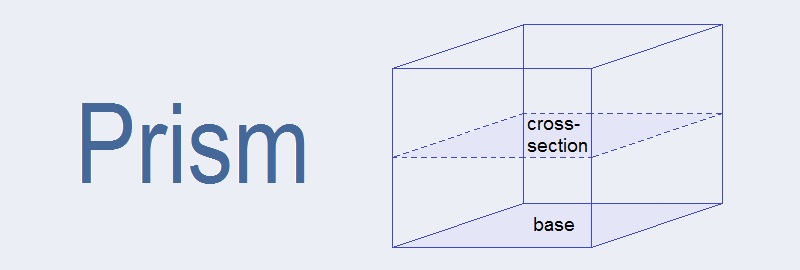# Prism## Prism

A solid object in which two opposite faces are congruent and parallel and its cross section part is congruent to its bases is called a prism. For example: cube, cuboid, cylinder etc are prisms. In the figure given below is a cuboid (prism).

The name of prism is determined with respect to its base.

#### Cuboid:

A rectangular prism is called a cuboid. A cuboid is also known as a rectangular parallelopiped.
Cross sectional area = Area of base
= l × b
Total surface area = Area of all 6 faces of the cuboid
= lb+lb+bh+bh+lh+lh
= 2lb + 2bh + 2lh
= 2(lb + bh + lh)
Volume = Area of base × height
= l × b × h

#### Cube:

A cuboid in which all three dimensions (length, breadth and height) are equal is called a cube.
Cross-sectional area = Area of base = l2
Total surface area = 6l2
Volume = l3

#### Cylinder:

A circular prism is called a cylinder.
Cross-sectional area = Area of base = Area of circle = πr2
Total surface area = Curved surface area + area of two bases (circles) = 2πrh + 2πr2
Volume = Area of base × height = πr2h

#### Triangular Prism:

A prism having its base a triangular shaped is called a triangular prism.
Cross-sectional area = Area of base triangle = A (formula according to the type of triangle)
Lateral surface area = Perimeter of Δ × height = Ph
Total surface area =  Lateral surface area + Area of 2 bases = Ph + 2A
Volume = Area of base triangle × height = Ah

### Workout Examples

Example 1: Find the area of cross-section, total surface area and volume of the given prism.
Solution: The given prism is a cuboid of,
Length (l) = 8cm
Height (h) = 12cm

Area of cross section = l × b
= 8cm × 6cm
= 48cm2

Total surface area = 2(lb+bh+lh)
= 2(8×6 + 6×12 + 8×12)
= 2(48 + 72 + 96)
= 2 × 216
= 432cm2

Volume = l × b × h
= 8cm × 6cm × 12cm
= 576cm3

Example 2: Find the cross-section area, total surface area and the volume of the given prism.
Solution: The given prism is a cube of,
Length (l) = 8cm

Area of cross section = l2
= (8cm)2
= 64cm2

Total surface area = 6l2
= 6 × (8cm)2
= 6 × 64cm2
= 384cm2

Volume = l3
= (8cm)3
= 512cm3

Example 3: Find the area of cross-section, total surface area and volume of the given prism.
Solution: The given prism is a cylinder of,
Height (h) = 12cm

Area of cross section = πr2
= 22/7 × 72
= 154cm2

Total surface area = 2πrh + 2πr2
= 2 × 22/7 × 7 × 12 + 2 × 22/7 × 72
= 528 + 308cm2
= 836cm2

Volume = πr2h
= 22/7 × 72 × 12
= 1848cm3

Example 4: Find the area of cross-section, total surface area and volume of the given prism.
Solution: The given figure is a triangular prism with right angled triangle base of,
base (b) = 4cm, perpendicular (p) = 3cm

Perimeter = 4cm + 3cm + 5cm = 12cm
Height of prism (h) = 10cm

Area of cross section (A) = Area of right angled triangle
= ½ × b × h
= ½ × 4 × 3
= 6cm2

Total surface area = Ph + 2A
= 12cm × 10cm + 2 × 6cm2
= 120cm2 + 12cm2
= 132 cm2

Volume = A × h
= 6cm2 × 10cm
= 60cm3

Example 5: Find the area of cross-section, total surface area and volume of the given prism.
Solution: Base is divided into two parts A1 and A2 as given below in the figure,
Area of cross section (A) = A1 + A2
= 5 × 3 + 4 × 2
= 15 + 8
= 23cm2

Total surface area = Perimeter of cross-section × h + 2 × cross-section area
= (5 + 3 + 3 + 4 + 2 + 7) × 8 + 2 × 23
= 24 × 8 + 46
= 192 + 46
= 238cm2

Volume = A × h
= 23cm2 × 8cm
= 184cm3

You can comment your questions or problems regarding the surface area and volume of prisms here.